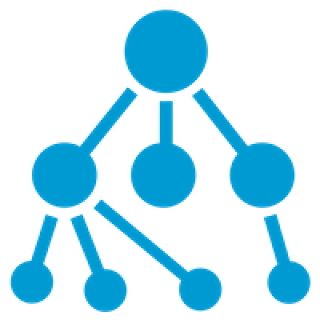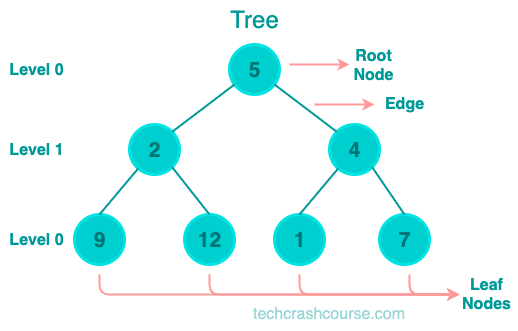# Tree Data Structure# Tree

A Tree is a nonlinear data structure. Unlike other linear data structures such as an array, stack, queue, and linked list, a tree depicts a hierarchical structure. The ordering information of a tree is not important.

The Tree data structure is widely used in computer science and software engineering.Here are some common Tree Terminologies
• Node: A node is an entity that contains a key or value and pointers to its child nodes.

• Edge: The connection between nodes is called an edge.

• Parent Node: The node which is a predecessor of a node is called the parent node of that node.

• Root Node: The topmost node of a tree without any parent nodes is known as the root. Every tree has a single root node.

• Leaf Node: The leaf node is a node with no children. It is the tree's last node. A tree may have several leaf nodes.

• Child Node: The node that immediately succeeds another node is referred to as the child node of that node.

• Sibling Nodes: Child node of the same parent node are called sibling nodes.

• Level of Node: A level is the number of parents that make up a specific node in the tree.

• Degree of Node: The degree of a node is the number of children it has. A node with no children is called a leaf node, and its degree is 0. A node with only one child is called a "unary" node, and its degree is 1. A node with two children is called a "binary" node, and its degree is 2.

• Depth of Node: The depth of a node in a tree is the number of edges on the path from the root node to that node. The depth of the root node is 0.

• Height of Node: The height of a node in a tree is the number of edges on the longest path from that node to a leaf node. If a node has no children, its height is 0. The height of a tree is the height of its root node.

• Height of Tree: The height of a tree is the height of its root node. It is the maximum depth of any node in the tree.

## Uses of Tree Data Structure

• Representing Hierarchical Data: The Tree data structure is an excellent way to represent hierarchical data, such as file systems, XML documents, and JSON objects.

• Searching and Sorting: Binary Search Trees (BST) are commonly used for searching and sorting data. A BST is a Tree where each node has two children at most, and the left child is smaller than the parent, while the right child is larger.

• Routing and Network Topology: The Tree data structure is used in networking to represent network topologies and routing tables.

• AI and Machine Learning: Decision Trees are used in AI and machine learning to model and predict outcomes based on input variables. Decision Trees are constructed by recursively splitting data based on the most significant variables.

## Benefits of Tree Data Structure

• Efficient Searching and Sorting: Binary Search Trees (BST) are an efficient data structure for searching and sorting data. The search and insert operations in a BST have a time complexity of O(log n), which is much faster than linear search algorithms in other data structures such as arrays and linked lists.

• Easy to Modify and Update: The Tree data structure is easy to modify and update. Adding or deleting a node in a Tree only requires modifying the links between the nodes, which can be done in constant time. In contrast, modifying an array or linked list requires shifting elements, which can be expensive.

• Hierarchical Representation: The Tree data structure is an excellent way to represent hierarchical data. The Tree structure provides a clear representation of parent-child relationships, which can be used to traverse and manipulate the data efficiently.

• Natural Recursion: Trees have a natural recursive structure that makes them well-suited for recursive algorithms. Many Tree algorithms use recursion to traverse the Tree and perform operations on the nodes.

## Tree Traversal

Tree traversal refers to the process of visiting all the nodes in a tree in a specific order. There are three common traversal methods: InOrder, PreOrder, and PostOrder.

### InOrder Traversal

In InOrder traversal, we visit the left subtree, then the current node, and then the right subtree. The algorithm is as follows:

1. Traverse the left subtree in InOrder.
2. Visit the root.
3. Traverse the right subtree in InOrder.
Here's an example of InOrder traversal
```     1
/   \
2     3
/ \   / \
4   5 6   7
```
InOrder traversal : 4 2 5 1 6 3 7

### PreOrder Traversal

In PreOrder traversal, we visit the current node, then the left subtree, and then the right subtree. The algorithm is as follows:

1. Visit the root.
2. Traverse the left subtree in PreOrder.
3. Traverse the right subtree in PreOrder.
Here's an example of PreOrder traversal
```     1
/   \
2     3
/ \   / \
4   5 6   7
```
PreOrder traversal : 1 2 4 5 3 6 7

### Post Order Traversal

In Post Order traversal, we visit the left subtree, then the right subtree, and then the current node. The algorithm is as follows:

1. Traverse the left subtree in Post Order.
2. Traverse the right subtree in Post Order.
3. Visit the root.
Here's an example of Post Order traversal
```     1
/   \
2     3
/ \   / \
4   5 6   7
```
PostOrder traversal: 4 5 2 6 7 3 1